# Geometry Expressions

## Geometry Expressions Books

Browse our range of books about Geometry Expressions.

Some are collections of lessons, suitable for photcopying and handing out to your class.

Others are extensive collections of examples.

All are available in printed or electronic form.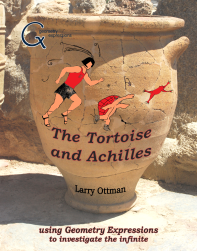### The Tortoise and Achilles:

##### using Geometry Expressions to investigate the infinite

by: Larry OttmanPhysical book (delivered by mail) \$24.95 + S&H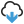PDF eBook (digital download) \$9.99

Calculus is the study of the infinite and since much of secondary mathematics is designed to prepare one for the study of calculus, wrestling with the ideas of the infinite, even if informally, is extremely important for a student's mathematical development. That is the purpose of this book.

Among the first to discuss these ideas was the Greek philosopher / mathematician Zeno, and not long after him, Archimedes came the closest to discovering Calculus without the tools of modern mathematics. Though none of Zeno's actual writings survive, Aristotle recorded accounts of Zeno's thoughts on the infinite, time and space in what have come to be known as Zeno's Paradoxes. One of those specifically involves the idea of a race in which a slower runner is given a head start and investigates the possibilities of the faster runner 'catching up'. This has come to be known as 'Achilles and the Tortoise'. These characters are our hosts as we use the modern software, Geometry Expressions, to investigate Archimedes methods, ideas of the infinite, and Zeno's Paradoxes in an introduction to Calculus, without using Calculus.### Calculus Explorations With Geometry Expressions

by: Irina Lyublinskaya, Valeriy Ryzhik, Ron Armontrout

The purpose of this book is to use Geometry Expressions software in order to facilitate and enhance the calculus syllabus by allowing students to ground calculus concepts in a geometric way. The 29 student explorations in this book cover the major topics of a standard course of calculus, and are completed with the help of the constraint-based dynamic software package, Geometry Expressions. Using Geometry Expressions in learning calculus, students have the opportunity to develop general investigation skills, make connections between geometric and algebraic representations of major calculus ideas, interpret analytic problems visually and geometric problems algebraically, and develop facility with using a computer to prove general mathematics statements. Geometry Expressions enables more extensive calculus investigation than is possible in a traditional course of calculus.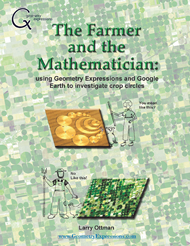### The Farmer and the Mathematician:

##### using Geometry Expressions and Google Earth to investigate crop circles

by: Larry Ottman

The typical student complaint about secondary mathematics is that it appears to have no practical application. The design and implementation of crop circles using center pivot irrigation provides us with an excellent example to see mathematics in action. By investigating these crop circles the lessons in this book utilize images from Google Earth, and a lighthearted scenario of a discussion between a farmer and a mathematician to introduce important mathematical concepts that are appropriate and adaptable for students in a range of courses from Pre-Algebra, Algebra, and Geometry, up through and including Calculus.### The Farmer and the Mathematician II:

##### more mathematical investigations using Geometry Expressions

by: Larry Ottman

This is Mr. Ottman's second book about a corny farmer and his mathematician friend. Our farmer uses a Center Pivot Irrigation System - those long stretches of wheeled scaffolding, sometimes up to a half-mile in length, rotating around a fixed point attached to his water source. This presents real world geometry problems concerning how to fit a circular or semi-circular irrigated plot into a polygonal (usually rectangular, sometimes triangular or even trapezoidal) field.

As in the first book, we demonstrate to the student that Mathematics is not just for calculating the price of produce in the grocery store. Here we have a fresh batch of examples of mathematics in action using our symbolic geometry software, Geometry Expressions, with images from Google Earth.

So join the Farmer and his mathematical instructor, Sophie, as they fly over Texas, investigating interesting geometrical ideas and stumbling over important mathematical concepts. The content is appropriate and adaptable for students in a range of courses from Algebra and Geometry up through and including Calculus.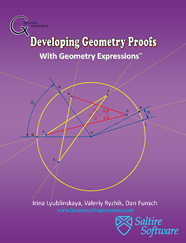### Developing Geometry Proofs with Geometry Expressions

by: Irina Lyublinskaya, Valeriy Ryzhik, Dan Funsch

We wrote this book with the hope to help teachers to engage their students in learning geometry and encourage them in their development of logical thinking. This book offers 43 interesting geometry problems that resulted from a collaboration of Russian and American educators. They reflect the content of the standard high school curriculum. Altogether these problems demonstrate the most important methods of geometry. The unique features of these problems include use of symbolic geometry software, Geometry Expressions. Each problem includes student black line master and detailed teacher notes with solutions, proofs, and steps for working with Geometry Expressions.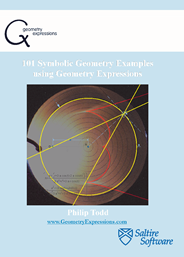### 101 Symbolic Geometry Examples Using Geometry Expressions

by: Philip Todd

This book comprises 101 examples of the use of Geometry Expressions in a variety of settings. In some cases, we give a simple model with little explanation. In other cases, there is some exposition backed up by Geometry Expressions models. Some examples make use of an algebra system in addition to Geometry Expressions, while many use Geometry Expressions stand-alone. Together, we hope, they give an indication of what the system can do, and provide a starting point for the reader to pursue his own discoveries.### 101 Conic Sections Examples using Geometry Expressions

by: Philip Todd

The goal of this book is to show you what you can do with the conic section capabilities of Geometry Expressions 2.0. The goal is not to teach you conic sections, though you might learn some interesting or arcane facts about them on the way. Neither is the goal to teach you how to use Geometry Expressions, though you might pick up a few pointers en route.### Connecting Algebra and Geometry through Technology:

##### applying Geometry Expressions in the Algebra 2 and Pre-Calculus classrooms

by: Jim Wiechmann

Geometry Expressions provides a playground where students can discover their own mathematics. They will begin to see mathematics as something that is created, not just a set of facts made up long ago. Once students take ownership of their mathematics, they will be more apt to "work productively and reflectively, with the skilled guidance of their teachers."

The units presented in this book are a jumping-off point for using Geometry Expressions in the classroom. Use the units to gauge the potential of this powerful software, and as a guide to applying Geometry Expressions in your own classroom. We trust that you will enjoy using the units and the software.### Function Transformations

by: Tim Brown

This unit is designed to familiarize students with the ideas of how various functions can be transformed, and the effect those transformations have on equations, graphs, and contextual situations. It specifically investigates three basic function types: those with parent functions y=x2, y=1/x, and y=sin(x). These function families were chosen because they are simple enough for students to readily understand, and also sophisticated enough to clearly demonstrate the effects of dilations and translations. The skills and principles to be learned apply to virtually all function families, and will give a solid foundation for more advanced studies in functions.### Exploring with Geometry Expressions in High School Mathematics

by: Ian Sheppard

From the back cover: "Ian Sheppard's book is based around a set of activities using the computer program Geometry Expressions. The author's passion to provide students with engaging learning opportunities is evident throughout. The activities embrace investigative approaches and seek to enhance student learning through discovery and the use of computer technology. Geometry Expressions has two features that add to the genre of dynamic interactive geometry software, constraints and symbolics. The activities and approach are designed to emphasize these additional features, constraints as an intuitive way to construct diagrams and symbolics as a link between algebra and geometry."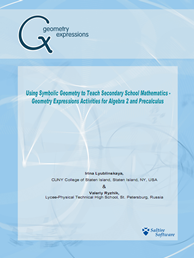### Using Symbolic Geometry to Teach Secondary School Mathematics:

##### Geometry Expressions activities for Algebra 2 and PreCalculus

by: Irina Lyublinskaya & Valeriy Ryzhik

From the back cover: Our goal was to use Geometry Expressions to develop a set of problems for second year algebra and/or precalculus courses addressing the topics of geometric transformations of functions and optimization. In this volume you will find eight interactive problems with different levels of difficulty. In each problem the main focus is on the development of the students' ability to recognize and make connections using multiple representations of the same object, such as geometric shape and function. By connecting geometric and algebraic representations the student develops a more thorough understanding of the problem.

### Learning Calculus with Geometry Expressions

by: L. Van Warren

The book is available in PDF format with accompanying Geometry Expressions files

Learn Calculus in a Week Instead of a Year! If 'a picture is worth a thousand words.' - an interactive animation is worth a thousand pictures! The new book, Learning Calculus with Geometry Expressions provides interactive examples for first year calculus.

What does this mean? Your student does not have to trudge through endless drills to master limits, derivatives and integrals. Study with our system, then go outside and play!

The introductory course presents a truly interactive approach to Calculus using Geometry Expression from Saltire Software. This dynamic, constraint based symbolic geometry software allows students to build geometry models and express them algebraically, both numerically and symbolically.

Students love to use technology in the classroom; the slides and examples contained in this course will motivate students and keep them engaged. And as an added bonus, each lesson begins with a vignette about an important mathematician. Van Warren's excellent instructions and beautiful Geometry Expressions examples present students with open-ended investigations which reinforce basic concepts and foster mathematical thinking.

Geometry Expressions eBook Bundle
by: Various AuthorsPDF eBook (digital download) \$85.95
The Geometry Expressions eBook bundle includes PDF versions of the following books:
• The Tortoise and Achilles: using Geometry Expressions to investigate the infinite
• Calculus Explorations With Geometry Expressions
• The Farmer and the Mathematician: using Geometry Expressions and Google Earth to investigate crop circles
• The Farmer and the Mathematician II: more mathematical investigations using Geometry Expressions
• Developing Geometry Proofs with Geometry Expressions
• 101 Symbolic Geometry Examples Using Geometry Expressions
• 101 Conic Sections Examples using Geometry Expressions
• Using Symbolic Geometry to Teach Secondary School Mathematics - Geometry Expressions Activities for Algebra 2 and PreCalculus
• Connecting Algebra and Geometry through Technology - Applying Geometry Expressions in the Algebra 2 and Pre-Calculus Classrooms
• Function Transformations
• Exploring with Geometry Expressions in High School Mathematics